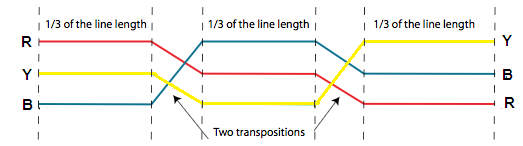# What is skin effect in transmission lines?A steady direct current flowing through the conductors is uniformly distributed over the whole cross-section of the conductor. However, an alternating current flowing through the conductor does not distribute uniformly, rather it tends to concentrate near the surface of the conductor as shown in the figure. This is known as skin effect in transmission line.

Thus, the tendency of an alternating current to concentrate near the surface of the conductor is known as skin effect in transmission line.

Due to skin effect, the effective area of the cross-section of the conductor through which current is to flow is reduced. This increases the resistance of conductor when carrying an alternating current. The cause of skin effect in transmission line is explained below:

Generally, a stranded conductor is used and each strand carries a small part of the current. The each conductor strand, near the center, is surrounded by a greater magnetic flux and hence has larger inductance than that strand near the surface. The high reactance (opposition) of inner stands restricts the flow of current in the center and causes it to flow near the surface of the conductor which produces skin effect.

## How to Reduce Skin Effect in Transmission Lines

The skin effect in transmission lines depends upon the following factors:

• Nature of material.
• The diameter of wire – The skin effect increases with the diameter of wire.
• The shape of wire – The skin effect is lesser for stranded conductor than the solid conductor.
• Frequency – The skin effect increases with the increase in frequency. When the frequency is zero (DC), there is no inductance and hence the current is uniformly distributed over the entire cross-section of the conductor. No skin effect. It is worthwhile to mention here that skin effect is negligible when the frequency is less (below 50 Hz) and conductor diameter is small (below 1 cm).

By utilizing this information properly, skin effect can be reduced considerably..

# What is proximity effect in transmission lines?

In the skin effect, the flux set up in the conductor itself was considered and it was assumed that there is no other current carrying conductor nearby. This flux produces a nonuniform distribution of current in the conductor called skin effect.

However, in the transmission lines, there are (one or two) current carrying conductors placed nearby and their magnetic flux links with the conductor under consideration. The effect of this flux is more in the nearer half of the conductor than the farther.

When the conductors carry currents in the opposite directions the fields set-up will tend to cause an increase in the current density in the adjacent portions whereas when the currents are in the same directions the current density is increased in the farther parts of the conductor. This effects the distribution of current in the conductor.

Thus, the flux set up by the nearby current carrying conductor effects the distribution of current in the conductor. This effect is called proximity effect in transmission lines. Hence, the proximity effect in transmission lines also results in the increase in resistance of the conductor.

## Transposition of Conductors in Transmission Lines

It has already been discussed that when conductors of a three-phase overhead line have symmetrically spaced the inductance and capacitance of the three phases are identical.

However, due to mechanical considerations, it is often desirable to space the conductors irregularly (unsymmetrical spacing). The inductance and capacitance of each phase will be different when conductors of a three-phase line are spaced irregularly. Rather, the apparent resistance of the conductors is also affected on account of the transfer of power between the phases, which occurs due to mutual inductance (proximity effect).

Thus, the result of this irregular spacing is that all the transmission line constants are different in three phases of the line. This makes different voltage drops in the three phases and results in different line voltages at the receiving end. This unbalancing effect on account of irregular spacing of conductors can be avoided by changing the position of conductors at regular distances. This is called transposition of conductors in transmission lines.

The process of changing the positions of the conductors (spaced unsymmetrically) of a three-phase overhead line at regular distances in order to make the transmission line constants of the three phases symmetrical, is called transposition of conductors in transmission lines.

In practice, the conductors have so transposed that each of them attains all the three positions for one-third of the total length of the line. The position of the three conductors is changed after every one-third of the length of the line.Thus for the first one-third length of the line, the position of the conductors is RYB, then over the next one-third the position is BRY and over the remaining one-third, the position is YBR. The transposition of conductors also reduces the inductive interference with the nearby communication circuits and thus reduces the disturbances.

## Advantages of transposition of conductors in transmission lines

Transposition of overhead line conductors of an unsymmetrical linehas the following advantages:

• It makes the transmission line constants of the three phases symmetrical which result in equal voltage drops in three phases. Hence equal line voltages are available at the receiving end.
• It reduces the disturbances in the neighbouring communication circuits.

Transmission System | All Posts

© https://yourelectricalguide.com/ skin effect in transmission line.